# MySQL 数据库入门学习

## 1. 前言

MySQL 很重要!很重要!很重要!

## 2. MySQL 基础知识

MySQL 经常和 “数据库” 连在一起读，这很容易对新手造成误解，认为 MySQL 就是一个数据库，其实不是这样，MySQL 是数据库的集合，MySQL 里面有很多数据库，那么数据是直接存在数据库里的吗？并不，数据库底下还有一个叫做数据表的存储单元，数据表里面才存储数据。打个比方，好比一座大图书馆，就是 MySQL，图书馆里面分南库，北库，这就是数据库，每个库里面的书都是放在书架上的，书架就是数据表，而放在书架上的很多本书，就是数据。这么说，读者应该理解了吧，所以按大小顺序排个序就应该是，MySQL > 数据库>数据表>数据，所以我们接下来的内容也是按照这个顺序来展开。

## 3. 进入 MySQL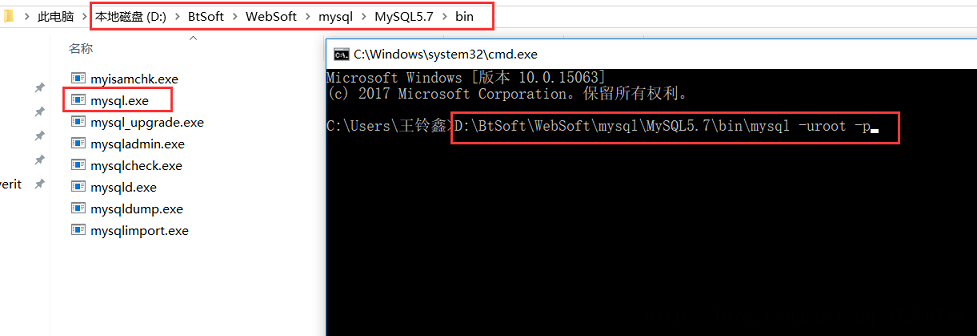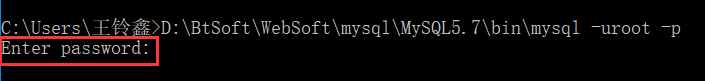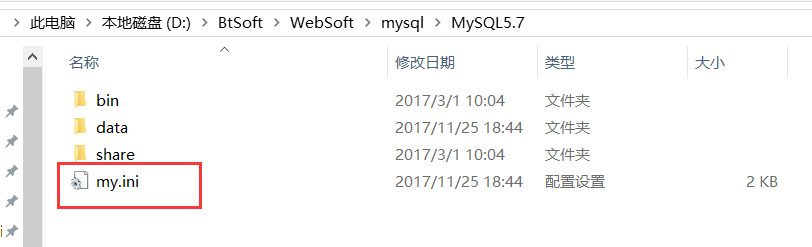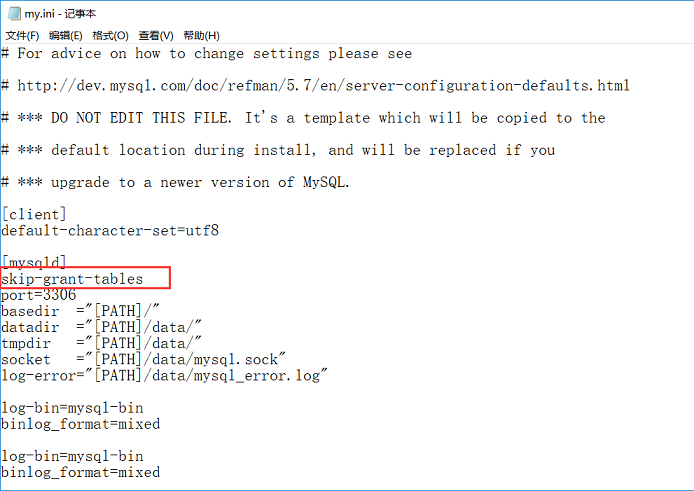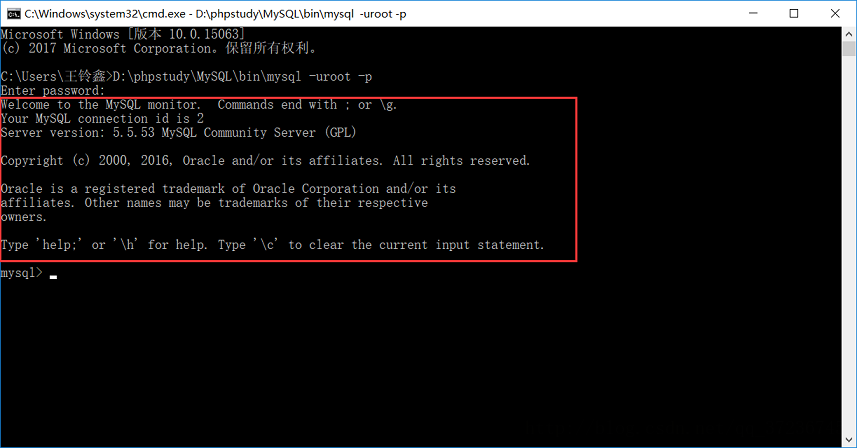## 4. 数据库的基本操作

1. 查询数据库

``show databases;``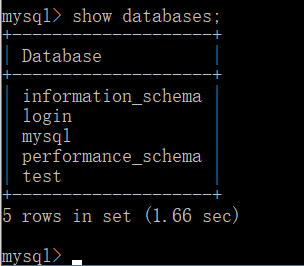2. 创建数据库

``create database XX(数据库名);``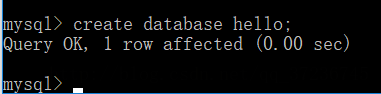``create database XX(数据库名) charset = (字符编码，例如utf8);``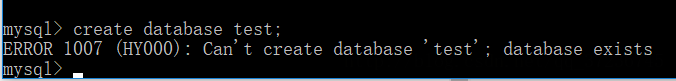果然，报错了，它告诉我，不能创建数据库 “test”，因为这个数据库已经存在了，所以我们创建数据库的时候判断一下，如果不存在就创建

``create database if not exists XX(数据库名); ``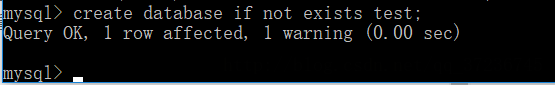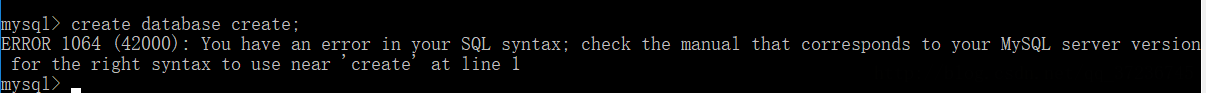``create database `XX`(数据库名);(注意，这里``是反引号，在键盘tab上面)``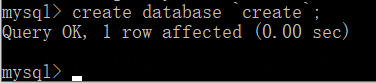3. 显示数据库信息

``show create database XX(数据库名);``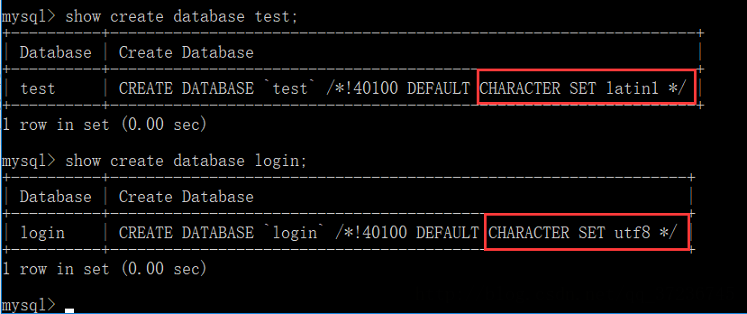4. 更改数据库的字符编码

``show create database charset = (字符编码，例如utf8);``

5. 删除数据库

``drop database XX(数据库名);``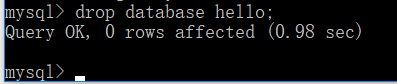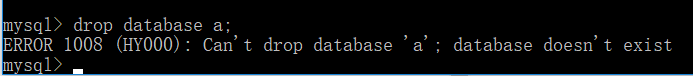``drop database if exists XX (数据库名字);    ``

5. 选择数据库

``use XX(数据库名);``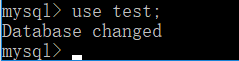## 5. 数据表的基本操作

1. 创建表

``````create table XX(表名)(

.......

);``````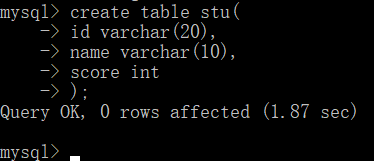``````数据类型：int 整型
tinyint 整型（0-256）
decimal 浮点型（总位数，小数位数） 例如 decimal(3,1)
char(X) 定长字符型 例如 char(10)
varchar(X) 可变长度字符型 例如varchar（10）
text 大段文本
binary 二进制（存储照片等）``````

``````null:空
not null:不为空
default 'XXXX'：默认值
auto_increment：自动增长
primary key：主键(主键的特点，不为空，不重复,一个表只能有一个主键，但是一个主键可以由多个列组成)``````

2. 查看所有表

``show tables;``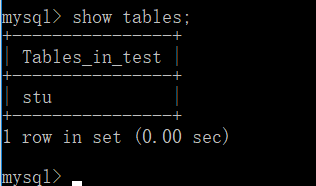3. 显示创建表

``````show create table XX(表名);
show create table XX(表名) \G;``````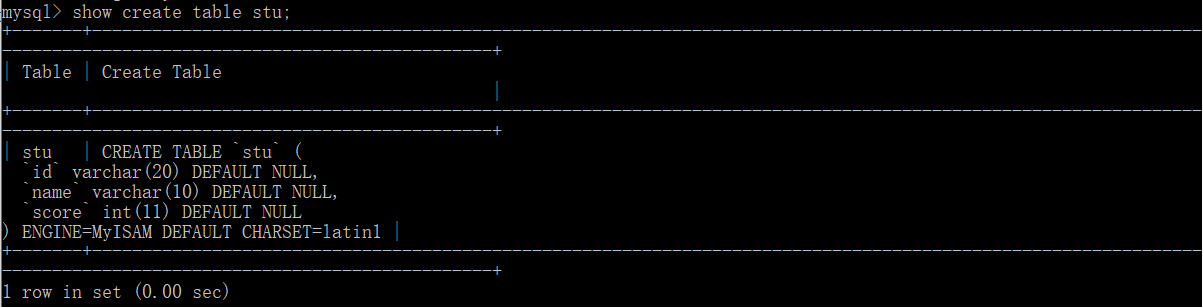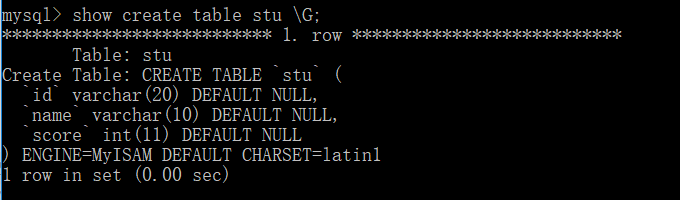4. 显示表结构

``describe(或desc) XX(表名);``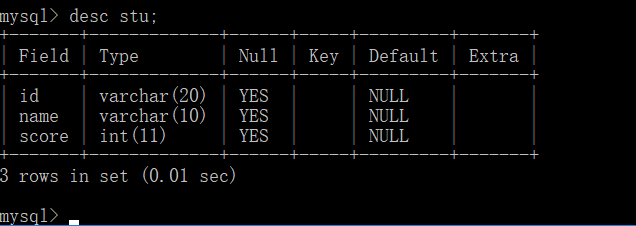5. 删除表（删除多个表）

``````drop table XX(表名);
drop table XX,XX,XX......; ``````

## 5. 数据的基本操作

1. 插入数据（增加）

``insert into XX（表名） (字段1,字段2,字段3......) values (值1,值2,值3.......);``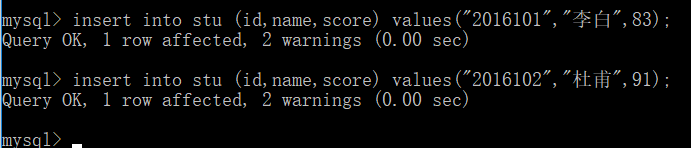2. 查询数据（查）

• 查询该表内的所有数据
``select * from XX（表名）;``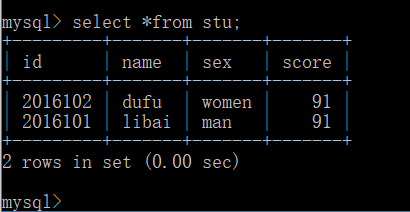• 查询该表内某字段的所有数据
``select 字段1,字段2,字段3......（或用"*"代替所有字段） from XX（表名）;``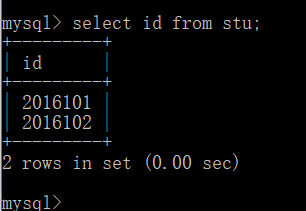• 查询某条件的所有数据
``select * from XX（表名）where 字段1 = 值1 and 字段2 = 值2 and ......;``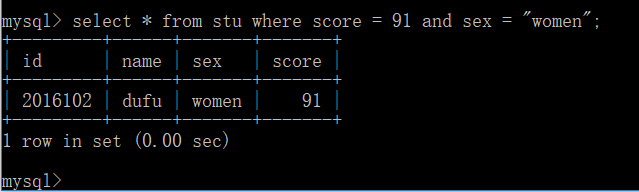``select * from stu where score > 90 or score < 60;``
• 由低到高排序
``select * from XX（表名） order by 字段x asc;（默认就是升序）``
• 由高到低排序
``select * from XX（表名） order by 字段x desc;``
• 按某条件排序
``select * from XX（表名） where 字段x = 值x oreder by 字段x asc(desc);``
• 取前 n 条数据
``select * from XX（表名） limit n;``
• 从第 n 条开始（起始位置是 0）取 m 条
``select * from XX（表名） limit n,m;``
• 查询某排序下的前 n 条数据
``select * from XX（表名） order by 字段x asc(desc) limit n;``

3. 删除数据（删）

``delete from XX（表名） where 字段x = 值x;``

4. 修改数据（改）

``````update XX（表名） set 字段1 = 值1,字段2 = 值2 where 条件;
update XX（表名） set 字段1 = 值1;``````

5. 聚合函数

• sum(); 求和 select sum(字段 x) from XX（表名）;
• avg(); 求平均值 select avg(字段 x) from XX（表名）;
• max(); 求最大值 select max(字段 x) from XX（表名）;
• min(); 求最小值 select min(字段 x) from XX（表名）;
• count(); 记录数 select count(字段 x) from XX（表名）;/select max(*) from XX（表名）;
• 在某字段条件下使用函数 select 函数名 () from XX（表名） where 字段 x = 值 x;

## 6. 结语

•